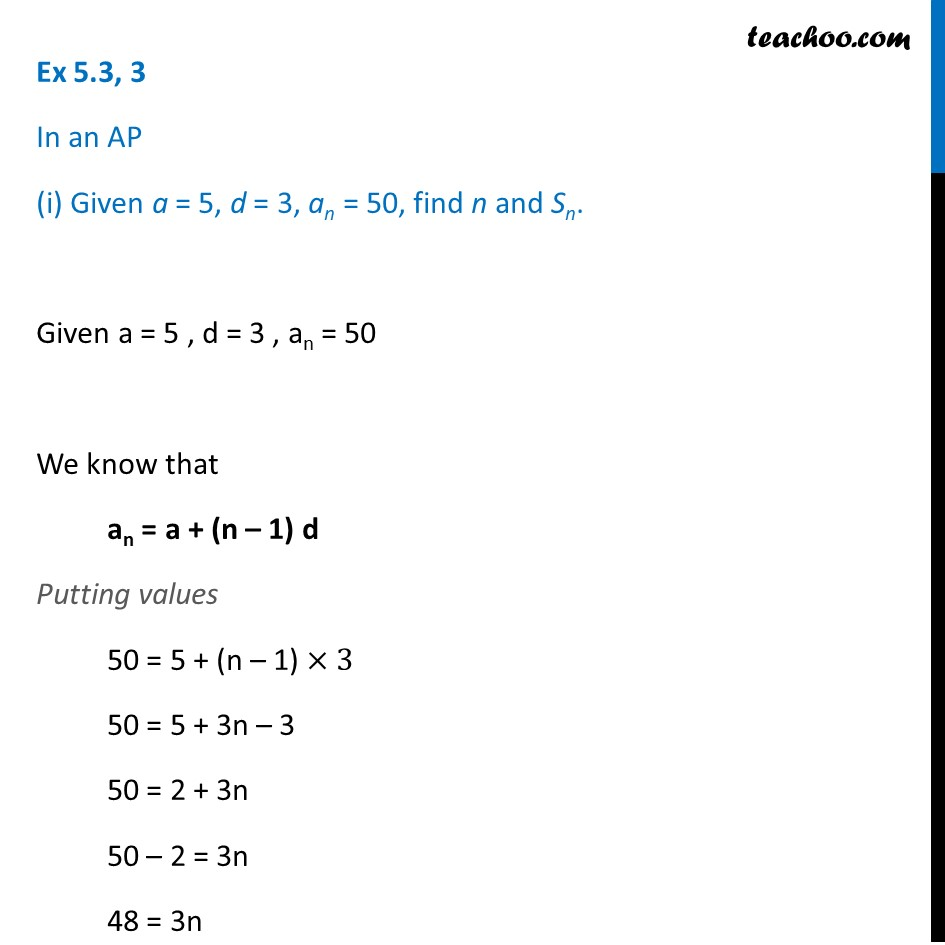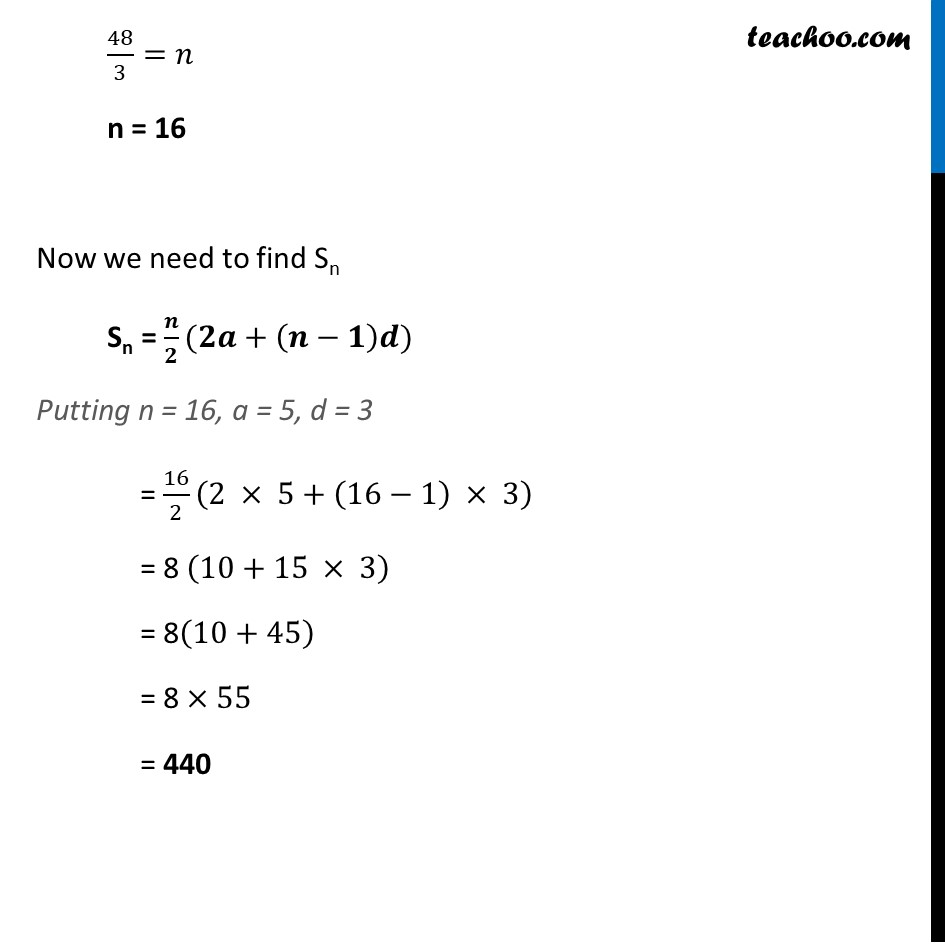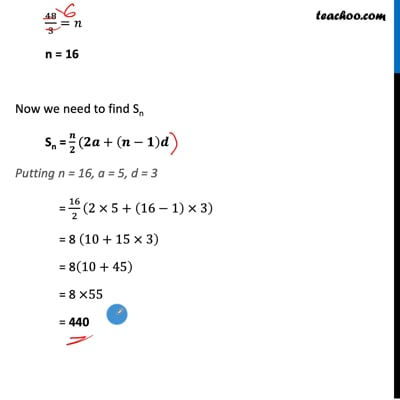Finding sum of n terms

Chapter 5 Class 10 Arithmetic Progressions (Term 2)
Concept wiseThis video is only available for Teachoo black users

### Transcript

Ex 5.3, 3 In an AP (i) Given a = 5, d = 3, an = 50, find n and Sn. Given a = 5 , d = 3 , an = 50 We know that an = a + (n – 1) d Putting values 50 = 5 + (n – 1) ×3 50 = 5 + 3n – 3 50 = 2 + 3n 50 – 2 = 3n 48 = 3n 48/3=𝑛 n = 16 Now we need to find Sn Sn = 𝒏/𝟐(𝟐𝒂+(𝒏−𝟏)𝒅) Putting n = 16, a = 5, d = 3 = 16/2 (2 × 5+(16−1) × 3) = 8 (10+15 × 3) = 8(10+45) = 8 ×55 = 440 So, answer is n = 7 and a = –8